# Two-Points Constraint Node

The Two-Points Constraint translates, rotates and scales a drawing to make it follow the pivots of the two pegs connected to it. Hence, it allows an animator to position an element (for example, a limb) by moving the two points at its extremities (the articulations).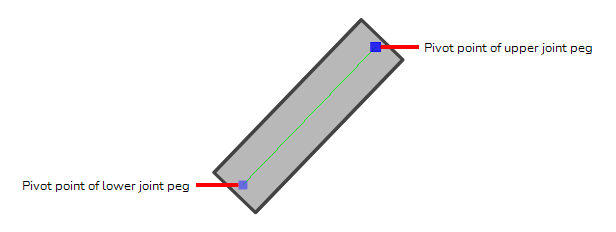Contrary to Three-Points Constraint and Multi-Points Constraint nodes, Two-Points Constraint can automatically stretch and squash the element they are connected to depending on the distance between the two points. This effect can be fine-tuned in the layer properties of the Two-Point Constraint node.

## Connection

The Two-Points Constraint node must be connected to two Peg nodes. The pivot point of each peg will define the resting position of one of the points used to transform the drawing connected under it. When one of the pegs is moved, the Two-Points Constraint will transform the drawing so that it follows the pivot point of that peg.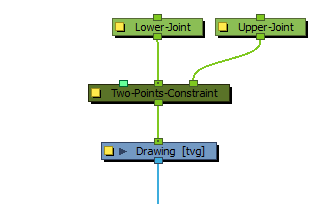NOTE The resting position of the points is based on the pivot point of the peg. The pivot point of a peg can be set by selecting the peg with the Translate, Rotate, Scaleor Skewtool, then clicking and dragging the pivot in the Camera view, or by entering its coordinates in the layer properties of the peg.

It is possible to rig multiple Two-Points Constraint nodes together in order to connect adjacent parts of a character rig by their articulation. For example, the upper and lower part of an arm or a leg could be rigged together in the following fashion, to allow the animator to manipulate them by their articulations: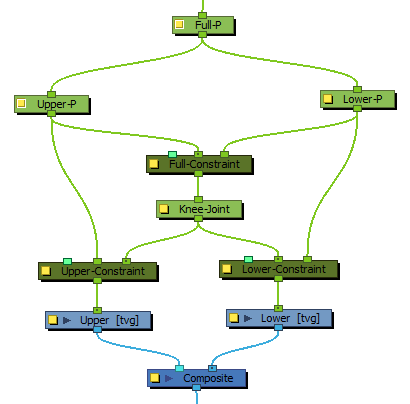Optionally, the leftmost input port of a Two-Points Constraint node can be connected to a Constraint-Switch, which can be used to reduce the effect of the Two-Points Constraint node on the element it is connected by setting its Active parameter between 0 and 100.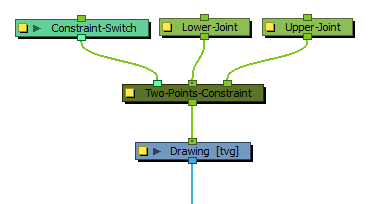TIP It is recommended to disable the Animate Using Animation Tools property of the element the Two-Points Constraint node is connected to. This will allow the animator to animate the Two-Points Constraint by selecting the element, then clicking and dragging near one of the two points using the Transform tool. The Two-Points Constraint will automatically select the closest point to the mouse cursor and move it.

## Controls

When the controls of the Two-Points Constraint are displayed, the position of the two points are displayed with blue squares linked by a green line.

These points are only a visual representation and cannot be manipulated.

## Properties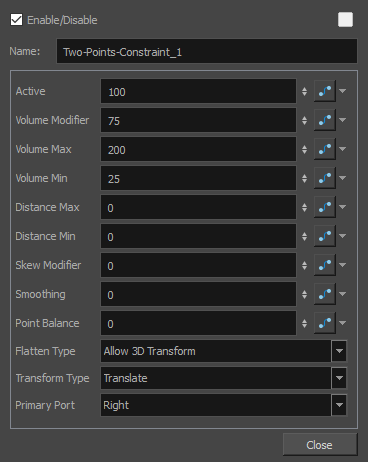Parameter Description Active The percentage of influence the constraint has on its child element. If set to 100, the element will follow the two points closely. If set to 0, the element will not be affected by the constraint. If a Transformation Switch node is connected to the Two-Points Constraint node, the Active parameters of the Transformation Switch and the Two-Points Constraint nodes will be multiplied together. Volume Modifier Defines how much the Two-Points Constraint node should preserve the width of the child element when the distance between the points causes it to stretch or squash. If set to 100, the width of the element will increase when squashed and decreased when stretched based on the Volume Max and Volume Min parameters. If set to 0, the element will always have the same width regardless of how much it is stretched or squashed. The value can be set to a number between 0 and 100 to reduce the effect of the volume modifications, to a number above 100 to increase it, or to a negative number to reverse the volume modification logic. Volume Max The maximum width of the element when squashed. This value represents a percentage of the element's original width. If set to 0, no minimum width will be applied. Volume Min The minimum width of the element when stretched. The value represents a percentage of the element's original width. If set to 0, no maximum width will be applied. Distance Min The minimum distance between the two points. If the points are brought closer together than their minimum distance, the element will stop transforming. This value represents a percentage of the distance between the two points when in their respective resting positions. Distance Max The maximum distance between the two points. If the points are brought farther from each other than their maximum distance, the element will stop transforming. This value represents a percentage of the distance between the two points when in their respective resting positions. Skew Modifier When set to 0, the element is rotated to follow the two points. If set to 100, the element will skew to follow the two points. If set between 0 and 100, the element will be transform with a combination of rotation and skewing to follow the two points. Smoothing When the two points are moved away from each other and reach their maximum distance, this value will adds an extra buffer of distance that the two points can reach before the element stops stretching. In this buffer, the way the element stretches is tapered, making it stop stretching smoothly instead of abruptly. This value represents a percentage of the distance between the two points when in their respective resting positions. Point Balance This parameter comes in effect when the two points are being moved at a distance exceeding their maximum distance. If set to 0, the element will stop stretching and remain locked against the primary point. If set to 100, the element will stop stretching and remain locked against the second point. If set to a value between 0 and 100, the element will be moved between both points, at a distance from the primary point that is proportional to this setting. Flatten Type This parameter defines what happens if one of the pegs defining the constraint points is moved on the Z-axis: Ignore 3D Transform: The element will be constrained to the vertical and horizontal position of the pegs, and will ignore their position on the Z axis. Allow 3D Transform: The element will be 3D-rotated to follow the position of the pegs in the 3D space. Project to Plane: The element will be constrained in 2D, but will be made to follow the perceived position of the points from the camera's point of view. This means the 3D position of the points will be projected on a 2D plane that is parallel to the camera. NOTE This projection is based on the position of the primary point peg on the Z-axis. Hence, if the two pegs are on a different position on the Z-axis, there may be a perceived offset between the secondary point and its effect on the element. Transform Type By default, only the position of the points is used to transform the element. Since those points are defined by pegs, these pegs can also be subjected to scale, angle and skew transformations that would be normally ignored by the Two-Points Constraint node. This parameter can be used to apply the other transformations done on the peg of the primary point on the element, rather than ignoring them. Translate: Ignores the transformations done on the primary peg, only applies the transformations required for the element to track the two points. Transform: Applies the transformations calculated by the Two-Points Constraint node to make the element to track the two points, then applies the scale, angle and skew values of the primary peg on top of them. Local Transform: Applies the scale, angle and skew values of the primary peg on the element, then applies the transformations calculated by the Two-Points Constraint node for the element to track the two points. Primary Port Defines which one of the ports, and hence, which one of the pegs the node is connected to, is used to define the primary point, leaving the other port for the secondary point. The primary point affects the other parameters in several ways: The Point Balance parameter ranges from 0 to 100, with 0 making the element balance towards the primary point and 100 making it balance towards the secondary point. When the Flatten Type is set to Ignore 3D Transform or Project to Plane, the position of the element on the Z-axis will be the one of the primary point. When the Flatten Type is set to Project to Plane, the position of the primary point on the Z-axis is used as the plane on which to project the 3D positions of the points. If the Transform Type is set to Transform or Local Transform, the scale, angle and skew transformations of the primary peg will be applied to the element.